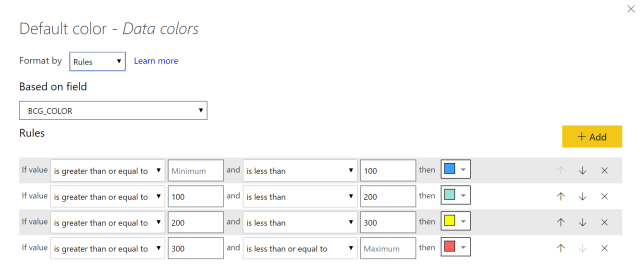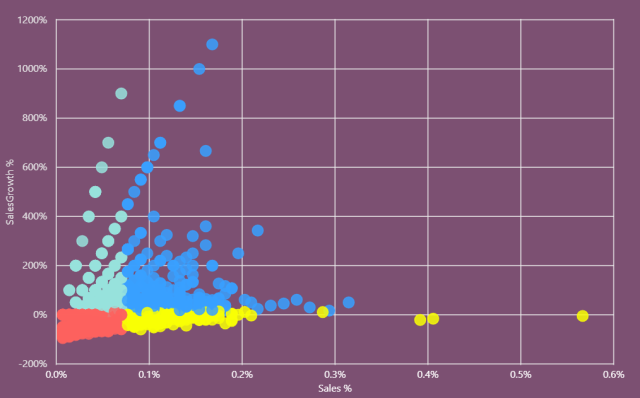# DAX波士顿矩阵可视化实现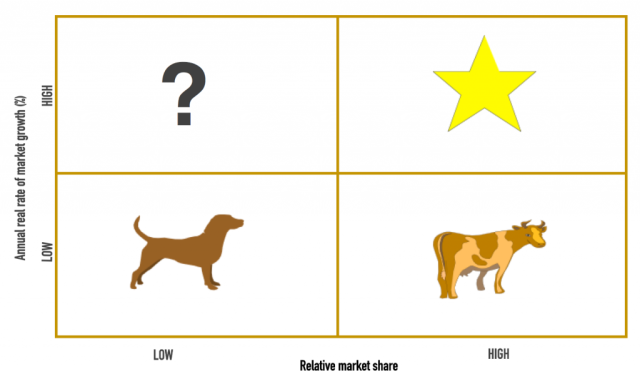``````--市场份额
Sales % =
DIVIDE(
sum(Orders[Quantity]),
CALCULATE(sum(Orders[Quantity]),
ALLEXCEPT(Orders,
'Date'[Month]))) -- 因市场增长率按上月环比计算，故此度量值需要能够被月份筛选
``````

``````--市场增长率
SalesGrowth % =
DIVIDE(
(SUM(Orders[Quantity])-
CALCULATE(SUM(Orders[Quantity]),
CALCULATE(sum(Orders[Quantity]),
)
``````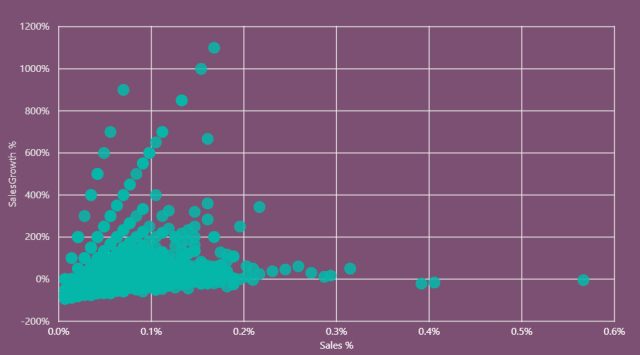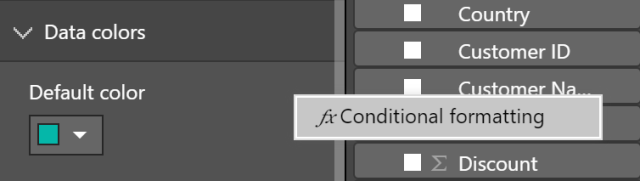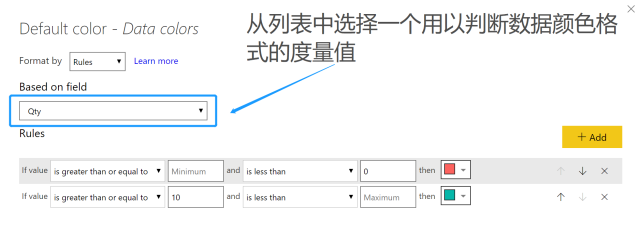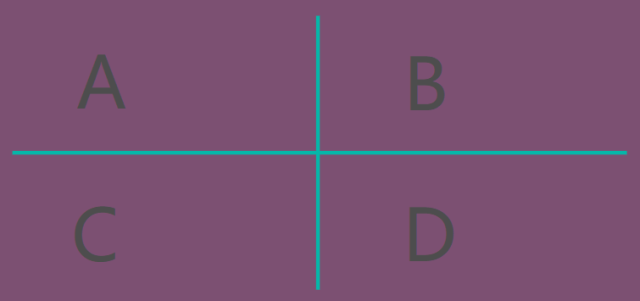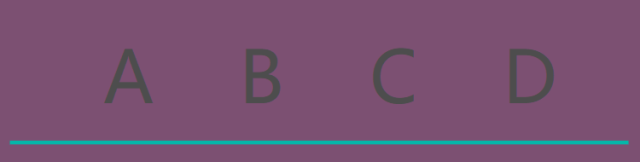``````BCG_COLOR =
var vt = SUMMARIZE('Date','Date'[Month])
var GrowthAvg =
CALCULATE(
AVERAGEX(vt,
'Orders'[SalesGrowth %]
),
all(Orders))
var SalesAvg =
CALCULATE(
AVERAGEX(Orders,
CALCULATE([Sales %],
ALLEXCEPT(Orders,Orders[Product Name]))),
ALLEXCEPT(Orders,'Date'[Month]))
var distance = ([SalesGrowth %]^2 + [Sales %]^2) ^ 0.5 + 1
var result =
if(and([SalesGrowth %]>=GrowthAvg,[Sales %]>=SalesAvg),"A",
if(and([SalesGrowth %]>=GrowthAvg,[Sales %]<SalesAvg),"B",
if(and([SalesGrowth %]<GrowthAvg,[Sales %]>=SalesAvg),"C","D")
)
)
return
if(distance = 0,BLANK(),
switch(result,
"A",LOG10(distance),
"B",LOG10(distance)+100,
"C",LOG10(distance)+200,
log10(distance)+300))
``````